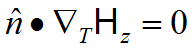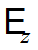﻿ Technical Notes > Applications in Electromagnetics > Waveguides > TE and TM Modes

# TE and TM Modes

Navigation:  Technical Notes > Applications in Electromagnetics > Waveguides >

# TE and TM ModesIn a homogeneously filled waveguide, there exist two sets of distinct modes. One set of modes has no magnetic field component in the propagation direction, and are referred to as Transverse Magnetic, or TM, modes.  The other set has no electric field component in the propagation direction, and are referred to as Transverse Electric, or TE, modes.  In either case, one member of (3.8) vanishes, leaving only a single variable and a single equation.  Correspondingly, equations (3.6) are simplified by the absence of one or the other field component.

In the TE case, we have, and the first of (3.8)
(3.9)The boundary condition at an electrically conducting wall is. Through (3.6), this implies,which is the Natural boundary condition of (3.9).

In the TM case, we have, and the second of (3.8)
(3.10).

The boundary condition at a metallic wall is, which requires that tangential components ofbe zero in the wall.  Sinceis always tangential to the wall, the boundary condition is the Dirichlet condition.

In the following example, we compute the first few TE modes of a waveguide of complex cross-section. The natural boundary condition allows an infinite number of solutions, differing only by a constant offset in the eigenfunction, so we add an integral constraint to center the eigenfunctions around zero.  Since all the material parameters are contained in the eigenvalue, it is unnecessary to concern ourselves with their values.  Likewise, the computation of the transverse field components are scaled by constants, but the shapes are unaffected.

#### Descriptor 3.1  Waveguide.pde

title "TE Waveguide"

select

modes = 4         { This is the number of Eigenvalues desired. }

variables

Hz

definitions

L = 2

h = 0.5               ! half box height

g = 0.01             ! half-guage of wall

s = 0.3*L             ! septum depth

tang = 0.1           ! half-width of tang

Hx = -dx(Hz)

Hy = -dy(Hz)

Ex = Hy

Ey = -Hx

equations

constraints   { since Hz has only natural boundary conditions,

we need to constrain the answer }

integral(Hz) = 0

boundaries

region 1

start(0,0)

natural(Hz) = 0

line to (L,0) to (L,1) to (0,1) to (0,h+g)

natural(Hz) = 0

line to (s-g,h+g) to (s-g,h+g+tang) to (s+g,h+g+tang)

to (s+g,h-g-tang) to (s-g,h-g-tang)

to (s-g,h-g) to (0,h-g)

to close

monitors

contour(Hz)

plots

contour(Hz) painted

vector(Hx,Hy) as "Transverse H" norm

vector(Ex,Ey) as "Transverse E" norm

end